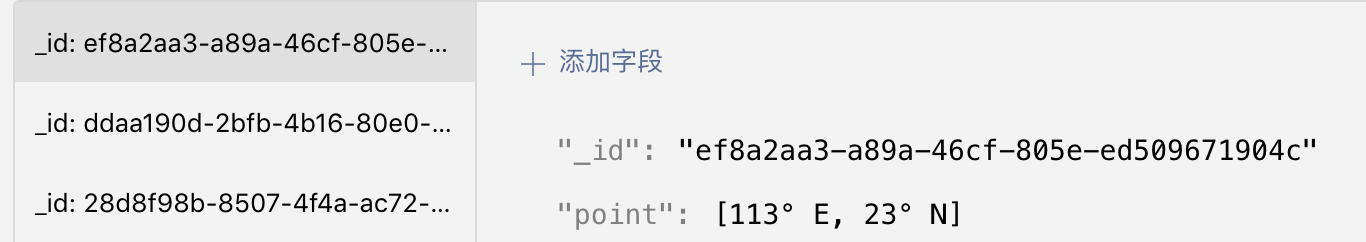# 置顶云开发数据库命令之地理位置查询精选热门

## geoNear：查询特定点附近的数据

### 数据查询实例``````const db = wx.cloud.database()
const _ = db.command
db.collection('items').where({
location: _.geoNear({
geometry: db.Geo.Point(113.323809, 23.097732),
minDistance: 1000,
maxDistance: 2000,
})
}).get()
``````

`minDistance` 则是距离中心点的最小距离，单位是米，所以这里将其设置为 1000。类似的，`maxDistance` 则是距离中心点的最大距离，单位也是米，所以这里将其设置为 2000。

## geoWithin：查询特定区域内的数据

### 数据查询实例``````const db = wx.cloud.database()
const _ = db.command
const { Point, LineString, Polygon } = db.Geo
db.collection('items').where({
location: _.geoWithin({
geometry: Polygon([
LineString([
Point(112, 22),
Point(112, 24),
Point(114, 24),
Point(114, 22),
Point(112,22)
])
]),
})
}).get()
``````

## geoIntersects：查询与特定区域相交的数据

`geoIntersects` 是用于查询所有数据中和给定数据相交的数据，我们可以将其用作判断某一些特定的点、线、面是否在一个特定区域内。举个例子，假设你已经有了用户当前的活动范围，比如某一条街道，那么你可以基于 `geoIntersects` 来构建一条线，并基于这条线查询，所有数据中，是否有数据与这个线相交，如果相交，则说明对应的数据点是在用户所在的街道上。你就可以将这个数据告诉用户，让用户去找这些点。

### 数据查询实例``````const db = wx.cloud.database()
const _ = db.command
const { Point, LineString, Polygon } = db.Geo
db.collection('items').where({
location: _.geoIntersects({
geometry: Polygon([
LineString([
Point(112, 22),
Point(112, 24),
Point(114, 24),
Point(114, 22),
Point(112,22)
])
]),
})
}).get()
``````

## 总结

2 个评论